Ch 1. Stress and Strain Multimedia Engineering Mechanics NormalStress Shear andBearing Stress NormalStrain Hooke'sLaw ThermalEffects IndeterminateStructures
 Chapter 1. Stress/Strain 2. Torsion 3. Beam Shr/Moment 4. Beam Stresses 5. Beam Deflections 6. Beam-Advanced 7. Stress Analysis 8. Strain Analysis 9. Columns Appendix Basic Math Units Basic Equations Sections Material Properties Structural Shapes Beam Equations Search eBooks Dynamics Fluids Math Mechanics Statics Thermodynamics Author(s): Kurt Gramoll ©Kurt GramollMECHANICS - CASE STUDY SOLUTION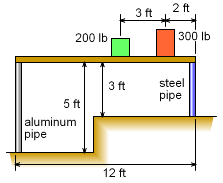Rack Storage Structure A storage rack is set up over a 2 foot rise in the floor in a warehouse. The rack spans a 12 foot space and is supported by two pipes, one at each end. The left pipe is an aluminum pipe (Eal = 10,000 ksi) and right pipe is a steel pipe (Est = 29,000 ksi). Both pipes have an outside diameter of 1/2" and a wall thickness of 1/16". The rack was constructed in winter at a temperature of 20oF and it was perfectly level. Later in the summer, when the temperature is at 125oF, the two packages are placed on the rack as shown. Thus the change in temperature is 105oF. Since it is critical that the shelf remains nearly level, the angle of rotation of the shelf needs to be calculated. This can be done by determining both the thermal expansion and the compression deflection due to the package loads of both pipes. Pipe Thermal Expansion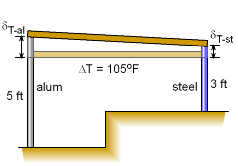Pipe Expansion After Temperature Change Since each pipe is a different length and made of different materials, their overall length changed due to the temperature change will be different.      δT-al = Lal αal (ΔT)             = (5 ft) (12 in/ft) (13×10-6/oF) (105o F)             = 0.08190 in      δT-st = Lst αst (ΔT)             = (3 ft) (12 in/ft) (6.5×10-6/oF) (105o F)             = 0.02457 in Pipe Compression Load and Deflection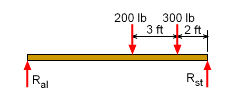Reactions at Pipe Supports Due to the packages on the rack, there will also be compression loads in the pipes which will shorten the pipes slightly. First, the compression loads in each pipe needs to be determined from basic static equilibrium equations.      ΣMst = 0      -Ral (12 ft) + (200 lb)(5 ft) + (300 lb)(2 ft) = 0      Ral = 133.33 lb      ΣFy = 0      -133.33 lb + 200 lb + 300 lb - Rst = 0      Rst = 366.67 lb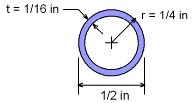Pipe Cross-Section Before finding the deflection, the cross-section area of the pipes needs to be known.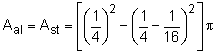= 0.08590 in2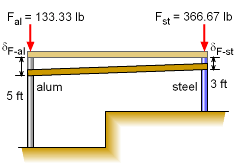Pipe Compression Deflection Due to Loads Recall, axial deflection is a function of the material length, stiffness, area and load. Now that the load is known, the deflection can be calculated for each pipe as,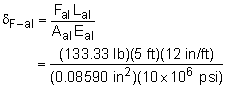= 0.009313 in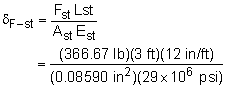= 0.005299 in Rack Rotation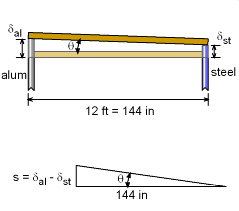Rotation Angle, θ The angle or rotation can now be determined from both the thermal and compression deflections.      δal = δT-al - δF-al = 0.8190 - 0.00931             = 0.08097 in      δst = δT-st - δF-st = 0.02457 - 0.005299             = 0.01927 in      s = δal - δst = 0.08097 in - 0.01927 in         = 0.06170 in The angle or rotation, θ, is      tanθ = s/144 = 0.06170/144 = 0.0004285 or      θ = 0.02455o This is a small angle, so the rack could be considered level.

Practice Homework and Test problems now available in the 'Eng Mechanics' mobile app
Includes over 400 problems with complete detailed solutions.
Available now at the Google Play Store and Apple App Store.